## 使用 MATLAB 探查器探查 MEX 函数

MATLAB Online™ 不支持探查器的图形界面。

### 生成 MEX 探查文件

1. 通过将配置对象属性 `EnableMexProfiling` 设置为 `true` 启用 MEX 探查。

您也可以将 `codegen``-profile` 选项结合使用。

MATLAB Coder App 中的等效设置是 Generate 步骤中的 Enable execution profiling

2. 生成 MEX 文件 `MyFunction_mex`

3. 运行 MATLAB 探查器，并查看探查摘要报告，该报告将在单独窗口中打开。

```profile on; MyFunction_mex; profile viewer;```

确保您没有更改或移动原始 MATLAB 文件 `MyFunction.m`。否则，探查器不会将 `MyFunction_mex` 视为探查对象。

• 通过将 `codegen``-test``-profile` 选项结合使用，一步生成 MEX 函数并对其进行探查。如果您以前打开过 MATLAB 探查器，请在结合使用这两个选项之前将其关闭。

`codegen MyFunction -test MyFunctionTest -profile`
• 通过在 App 的 Verify 步骤中选择 Enable execution profiling 来探查 MEX 函数。如果您以前打开过 MATLAB 探查器，请在执行此操作之前将其关闭。

### 示例

```function [y] = MyFunction(A,B) %#codegen % Generated code uses row-major representation of matrices A and B coder.rowMajor; length = size(A,1); % Summing absolute values of all elements of A and B by traversing over the % matrices row by row sum_abs = 0; for row = 1:length for col = 1:length sum_abs = sum_abs + abs(A(row,col)) + abs(B(row,col)); end end % Calling external C function 'foo.c' that returns the sum of all elements % of A and B sum = 0; sum = coder.ceval('foo',coder.ref(A),coder.ref(B),length); % Returning the difference of sum_abs and sum y = sum_abs - sum; end```

```#include <stdio.h> #include <stdlib.h> #include "foo.h" double foo(double *A, double *B, double length) { int i,j,s; double sum = 0; s = (int)length; /*Summing all the elements of A and B*/ for(i=0;i<s*s;i++) { sum += A[i] + B[i]; } return(sum); }```

```#include "rtwtypes.h" double foo(double *A, double *B, double length);```

`foo.c` 返回变量 `sum`，它是 `A``B` 的所有元素的总和。函数 `foo.c` 的性能与矩阵 `A``B` 以行优先布局还是列优先布局表示无关。`MyFunction` 返回 `sum_abs``sum` 的差值。

1. `MyFunction` 启用 MEX 探查并生成 MEX 代码。对两个大型随机矩阵 `A``B` 运行 `MyFunction_mex`。查看探查摘要报告。

```A = rand(20000); B = rand(20000); codegen MyFunction -args {A,B} foo.c foo.h -profile profile on; MyFunction_mex(A,B); profile viewer;```

将打开单独的窗口，显示探查摘要报告。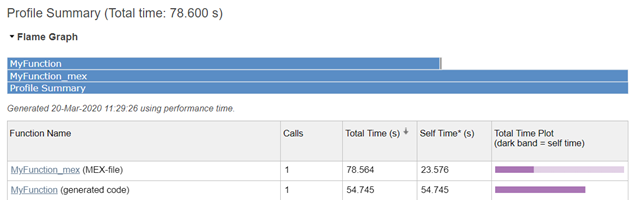探查摘要报告显示 MEX 文件及其子文件（为原始 MATLAB 函数生成的代码）的总时间和自用时间。

2. 在 Function Name 下，点击第一个链接查看为 `MyFunction` 生成的代码的探查详细信息报告。您可以查看耗时最多的代码行：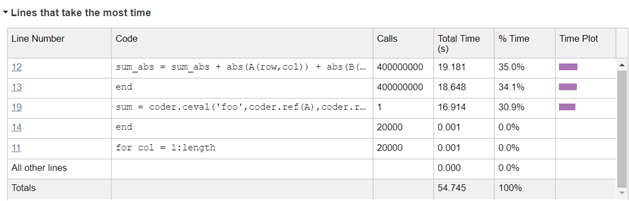3. 调用 `coder.ceval` 的代码行需要很长时间（16.914 秒）。此代码行的执行时间很长，因为 `coder.ceval` 将矩阵 `A``B` 的表示从行优先布局转换为列优先布局，然后将其传递给外部 C 函数。您可以通过在 `coder.ceval` 中使用附加参数 `-layout:rowMajor` 来避免此转换：

`sum = coder.ceval('-layout:rowMajor','foo',coder.ref(A),coder.ref(B),length);`
4. 使用修改后的 `MyFunction` 再次生成 MEX 函数和探查。

```A = rand(20000); B = rand(20000); codegen MyFunction -args {A,B} foo.c foo.h -profile profile on; MyFunction_mex(A,B); profile viewer;```
`MyFunction` 的探查详细信息报告显示，调用 `coder.ceval` 的代码行现在只需 0.653 秒：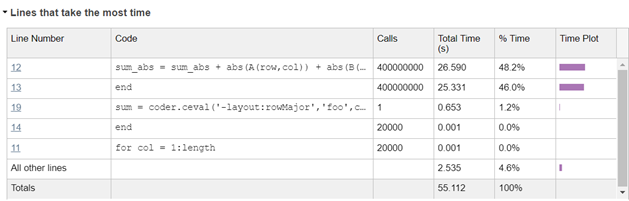### 折叠表达式对 MEX 代码覆盖率的影响

```function y = MyFoldFunction %#codegen a = 1; b = 2; c = a + b; y = 5 + coder.const(c); end```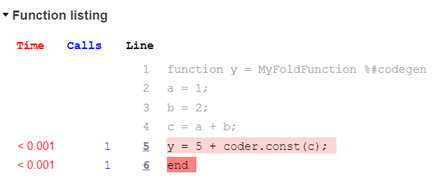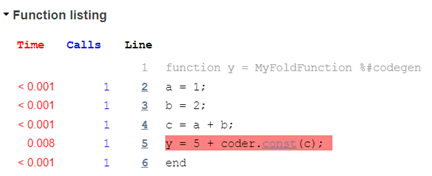## 支持